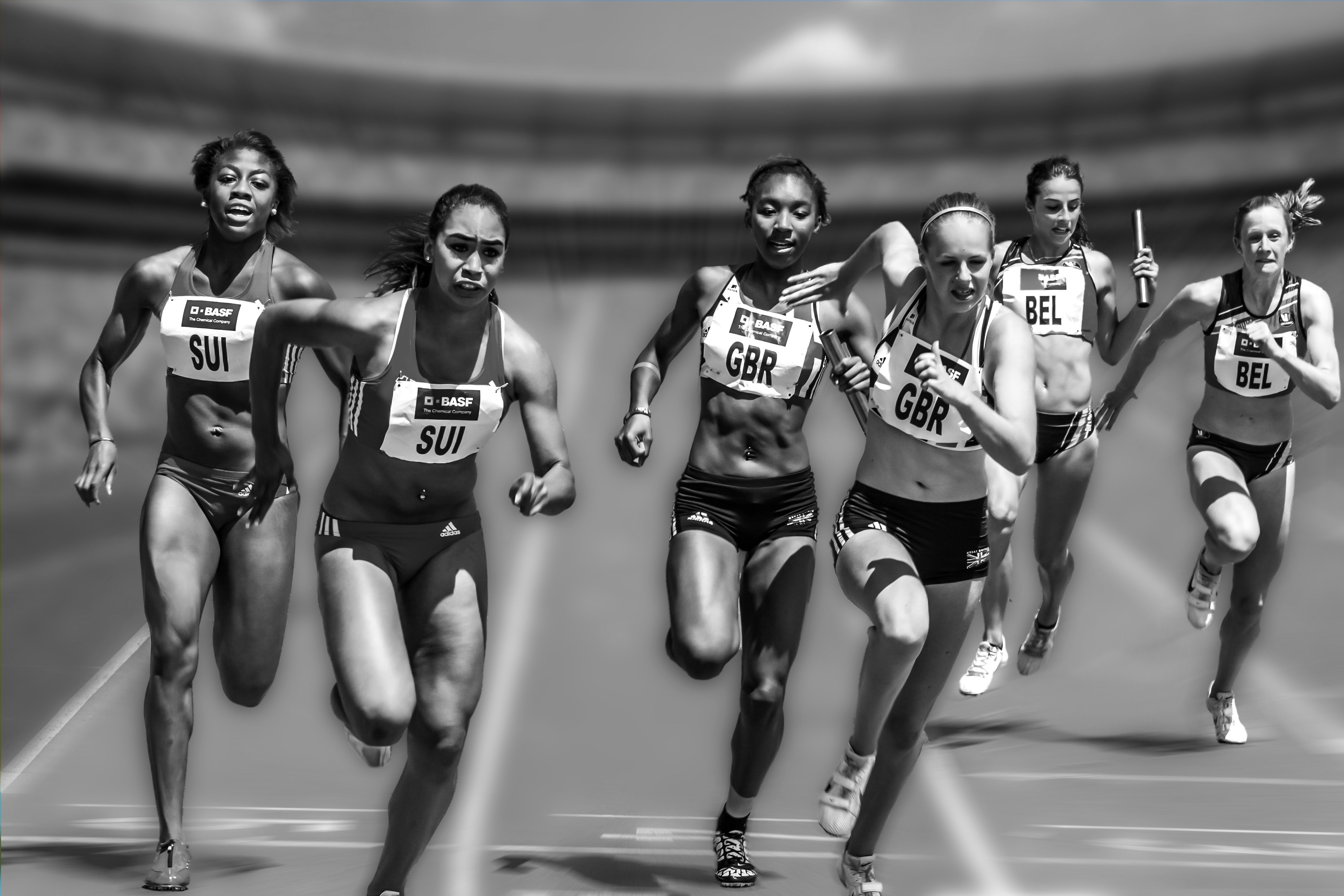# Momentum – Definition, Meaning, and Formula

Last updated on April 16th, 2021 at 01:01 pm

In this post, we will cover the definition and formula of Momentum (Linear Momentum). We will also discuss what it actually means in physics.

## Momentum Definition | Linear Momentum

By Momentum, we mean inertia in motion. More specifically, Linear Momentum or Momentum is defined as the product of the mass and velocity of an object. It’s designated with sign p.

We will use this sign p now onwards in this topic instead of the full name of the quantity we are discussing

## Momentum meaning or interpretation in physics

While playing some sort of body-contact game, if a heavy (with more mass) but slow moving person(less velocity) pushes us, we tend to move from our initial position.

Similarly if a lighter person (less mass) comes with higher velocity and pushes us we may face the same net effect and again begin to move.

In physics, to understand this combined motion effect of mass and velocity, this quantity p comes into picture.

## Momentum formula or equation

The formula or equation is written as:

p = m v

where m is the mass of the object and v is its velocity.

From the example in the beginning of this topic and the above equation, we see that p is directly proportional to the mass and velocity of an object.

As a result a heavy but slowly moving object may have a p equal (in magnitude) to that of a light but fast moving object.

## SI Unit

SI unit of momentum is Kg meter/second.

CGS unit of momentum is gm centimeter/second.

## Momentum is Vector or Scalar?

Because the velocity v is a vector quantity with both magnitude and direction, momentum(p) is also a vector quantity.

As momentum is a vector, the total momentum of a system consisting of objects is the vector sum of the momenta of all the objects in that system.

See also  Vertical motion when a ball is thrown vertically upward with derivation of equations

## Related study: Next 2 Parts

In the next parts of this discussion, we will cover impulse first. And then find out how momentum is related to impulse. We will cover how Newton’s second law and equation of force come into play to derive the relation between p and impulse.

*Next 2 Parts of this topic – read these as well* Links below

Impulse

Impulse Momenta Theorem

Scroll to top
error: physicsTeacher.in